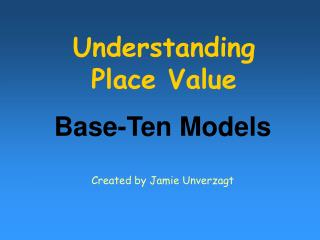DownloadDownload PresentationUnderstanding Place Value

# Understanding Place Value

Download Presentation## Understanding Place Value

- - - - - - - - - - - - - - - - - - - - - - - - - - - E N D - - - - - - - - - - - - - - - - - - - - - - - - - - -
##### Presentation Transcript

1. Understanding Place Value Base-Ten Models Created by Jamie Unverzagt

2. I have 1 hundred and 1 ten. What number am I?

3. I have 1 hundred and 1 ten. What number am I? 1 hundred 1 ten 0 ones

4. I have 1 hundred and 1 ten. What number am I? 1 1 0

5. I have 2 tens and 8 ones. What number will I be if you add 4 tens? 61 65 62 66 63 67 64 68 10 20 30 40 50 60

6. I have 1 hundred, 5 tens, and 2 ones. What number will I be if you take away 5 tens?

7. I have 1 hundred, 5 tens, and 2 ones. What number will I be if you take away 5 tens? 1 hundred 0 tens 2 ones

8. I have 1 hundred, 5 tens, and 2 ones. What number will I be if you take away 5 tens? 102

9. I have 1 hundred, 5 tens, and 2 ones. What number will I be if you take away 5 tens? 102

10. I have 1 hundred, 5 tens, and 2 ones. What number will I be if you take away 5 tens? 102

11. I am the greatest even number that has 3 tens. What number am I? What’s the greatest number of ones I can have and still be even? What’s the greatest number of hundreds I can have? How many tens?

12. I am the greatest even number that has 3 tens. What number am I? 938 9hundreds 3 tens 8 ones

13. I have 23 ones. What number am I? 2 tens 3 ones

14. I have a dozen tens. What number am I? 1 hundred 2 tens 0 ones

15. Can you show 23 with your blocks? Let’s write this number in expanded form. What is the value of each group of blocks? 1 3 2 10 20 2 tens is 20. 3 ones is 3. 20+3=23

16. Can you show 145 with your blocks? Let’s write this number in expanded form. What is the value of each group of blocks? 1 4 2 5 3 100 10 20 30 40 1hundred is 100. 4 tens is 40. 5 ones is 5. 100+40 + 5=145

17. Use Your Noodle Questions: • How many different ways can you show 21? • I have 3 blocks. The blocks can be of any value. What could one total value be? What are all the possible values I could have? Noodle Questions are from Creative Publications 20 Thinking Questions for Base Ten Blocks (Grades 2-3). ISBN 1-56107-792-5

18. Great Job!